1564383631

# Build up a Neural Network with python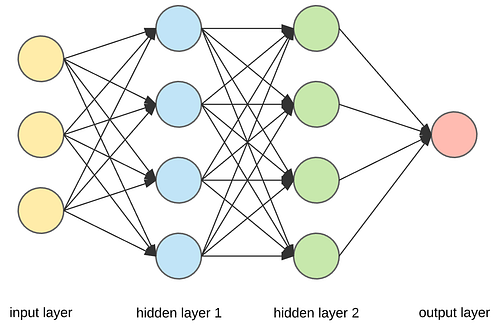Figure 1: Neural Network

Although well-established packages like Keras and Tensorflow make it easy to build up a model, yet it is worthy to code forward propagation, backward propagation and gradient descent by yourself, which helps you better understand this algorithm.

# Overview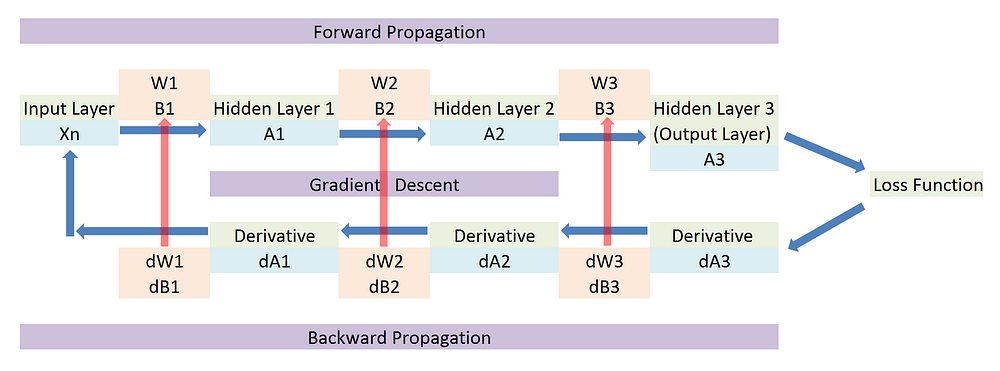Figure 2: Overview of forward propagation and backward propagation

Figure above shows how information flows, when a neural network model is trained. After input Xn is entered, a linear combination of weights W1 and bias B1 is applied to Xn. Next, an activation function is applied to have a non-linear transformation to get A1. Then A1 is entered as input for next hidden layer. Same logic is applied to generate A2 and A3. The procedure to generate A1A2 and A3 is called forward propagation. A3, also regarded as output of the neural network, is compared with independent variable y to calculate cost. Then derivative of cost function is calculated to get dA3. Take a partial derivative of dA3 for W3 and B3 to get dW3 and dB3. Same logic is applied to get dA2, dW2, dB2, dA1, dW1 and dB1. The procedure to generate a list of derivatives is called backward propagation. Finally gradient descent is applied and parameters are updated. Then a new round iteration starts with updated parameters. The algorithm will not stop until it converges.

# Create Testing Data

Create a small set of testing data to verify functions created.

```#############################################################
# Create test data
#############################################################
X = np.array([[1,0],[1,-1],[0,1]])
y = np.array([1, 1, 0])
```

# Initialize Parameters

In the stage of parameter initialization, weights are initialized as random values near zero. “If weights are near zero, then the operative part of sigmoid is roughly linear, and hence the neural network collapses into an approximately linear model.”  The gradient of sigmoid function around zero is big, so parameters can be updated rapidly by using gradient descent. Do not use zero and large weights, which leads to poor solutions.

```#########################################
# Step 1: Initialize Parameters
#########################################
def initialize_parameters(layer_dim):
np.random.seed(100)
parameters = {}
Length = len(layer_dim)
```for i in range(1,Length):
parameters['w'+str(i)]=np.random.rand(layer_dim[i],layer_dim[i-1])*0.1
parameters['b'+str(i)]=np.zeros((layer_dim[i],1))

return parameters
```
Test
test_parameters=initialize_parameters([2,2,1])
print(test_parameters)
```

I manually calculated one iteration training of neural network in Excel, which help you to verify accuracy of functions created at each step. Here is the output of parameter initialization on testing data.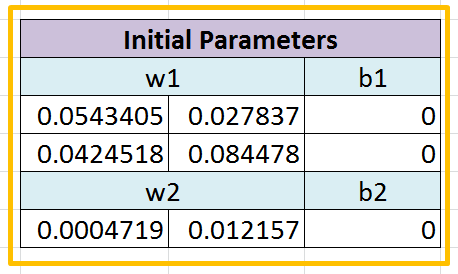Table 1: Parameters Initialization Testing Result

# Forward Propagation

In the neural network, inputs Xn is entered and information flows forward through the whole network. The inputs Xn provide the initial information that propagates up to hidden units at each layer and finally produces prediction. This procedure is called forward propagation. Forward propagation consists of two steps. First step is the linear combination of weight and output from last layer (or Inputs Xn) to generate Z. Second step is to apply activation function to have a nonlinear transformation.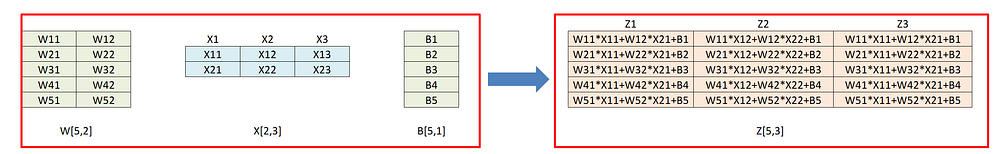Table 2: Matrix Calculation in forward propagation

In the first step, you need to pay attention to the dimension of input and output. Suppose you have an input matrix X with dimension of [2, 3] and one column in the matrix represents a record. There are 5 hidden units in the hidden layer, so the dimension of weight matrix W is [5, 2]. The dimension of bias B is [5, 1]. By applying matrix multiplication, we can get output matrix Z with dimension of [5, 3]. Details of calculation can be seen in the table above.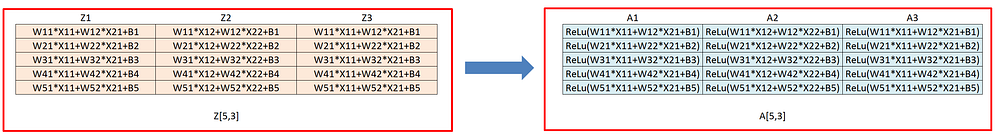Table 3: How activation is applied in forward propagation

Table above shows that how activation function is applied to each component of Z. The reason to use activation function is to have a nonlinear transformation. Without activation function, no matter how many hidden layers model has, it is still a linear model. There are several popular and commonly used activation functions, including ReLU, Leaky ReLU, sigmoid, and tanh function. Formulas and figures for those activation functions are shown below.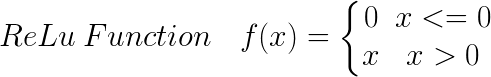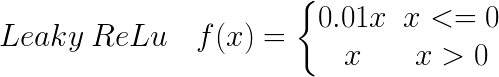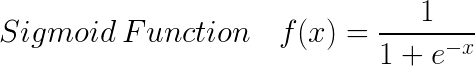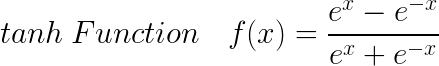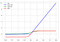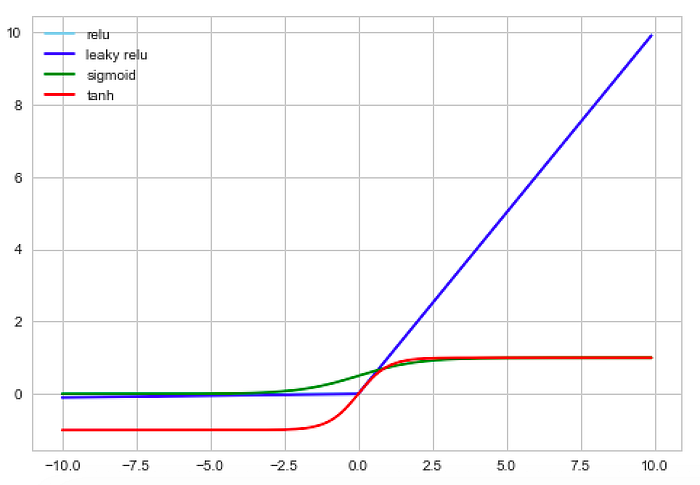Figure 3: Activation Function

```#######################################
Step 2: Forward propagation
#######################################
def sigmoid(x):
return(1/(1+np.exp(-x)))
def relu(x):
return(np.maximum(0,x))
def single_layer_forward_propagation(x,w_cur,b_cur,activation):
# Step 1: Apply linear combination
z=np.dot(w_cur,x)+b_cur
# Step 2: Apply activation function
if activation is ‘relu’:
a = relu(z)
elif activation is ‘sigmoid’:
a = sigmoid(z)
else:
raise Exception(‘Not supported activation function’)
```return z,a
```
Test
test_z,test_a=single_layer_forward_propagation(np.transpose(X),test_parameters[‘w1’],test_parameters[‘b1’],‘relu’)
print(test_z)
print(test_a)
def full_forward_propagation(x,parameters):
# Save z, a at each step, which will be used for backpropagation
caches = {}
caches[‘a0’]=X.T
```A_prev=x
Length=len(parameters)//2

# For 1 to N-1 layers, apply relu activation function
for i in range(1,Length):
z, a = single_layer_forward_propagation(A_prev,parameters['w'+str(i)],parameters['b'+str(i)],'relu')
caches['z' + str(i)] = z
caches['a' + str(i)] = a
A_prev = a

# For last layer, apply sigmoid activation function
z, AL = single_layer_forward_propagation(a,parameters['w'+str(Length)],parameters['b'+str(Length)],'sigmoid')
caches['z' + str(Length)] = z
caches['a' + str(Length)] = AL

return AL, caches
```
Test
test_AL,caches=full_forward_propagation(X.T,test_parameters)
print(test_AL)
```

First, you need to define sigmoid and ReLU function. Then create function for single layer forward propagation. Finally, functions created in the previous step is nested into the function called full forward propagation. For simplicity purpose, ReLU function is used in the first N-1 hidden layers and sigmoid function is used in the last hidden layer (or output layer). Note that in the case of binary classification problem, sigmoid function is used; in the case of multiclass classification problem, softmax function is used. Save Zand A calculated in each hidden layer into caches, which will be used in backward propagation.

Here is the function output on testing data.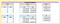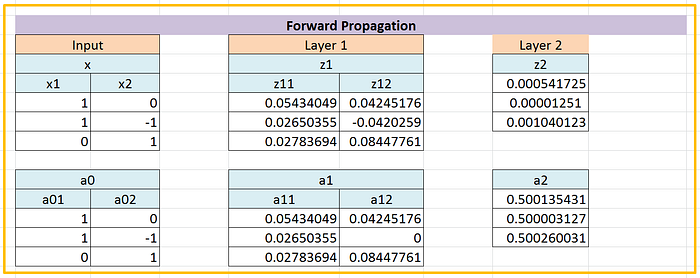Table 4: Forward Propagation Testing Result

# Cost Function

The output of forward propagation is the probability of binary events. Then the probability is compared with response variable to calculate cost. Cross entropy is used as cost function in the classification problem. Mean square error is used as cost function in the regression problem. Formula for cross entropy is shown below.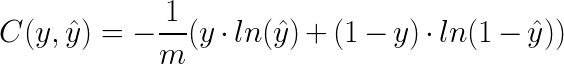```#########################################
Step 3: Cost function
#########################################
def cost_function(AL,y):
m=AL.shape
cost = (-1/m) * np.sum(np.multiply(y,np.log(AL)) + np.multiply((1-y),np.log(1-AL)))
# Make sure cost is a scalar
cost = np.squeeze(cost)
```return cost
```
Test
test_cost=cost_function(test_AL,y)
print(test_cost)
def convert_prob_into_class(AL):
pred = np.copy(AL)
pred[AL > 0.5]  = 1
pred[AL <= 0.5] = 0
return pred
def get_accuracy(AL, Y):
pred = convert_prob_into_class(AL)
return (pred == Y).all(axis=0).mean()
Test
test_y_hat=convert_prob_into_class(test_AL)
test_accuracy = get_accuracy(test_AL,y)
```

Here is the function output on testing data.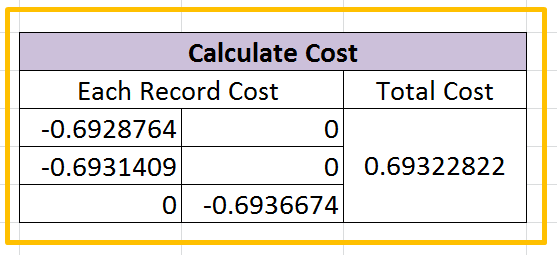Table 5: Cost Function Testing Result

# Backward Propagation

During training, forward propagation can continue onward until it produces a cost. The backward propagation is to calculate the derivatives of cost function and flow all information back to each layer, using chain rule in the calculus.

Suppose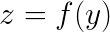and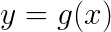Then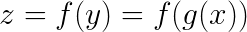Chain rule then states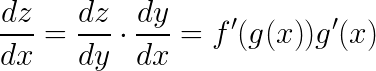The derivatives for activation functions are shown below.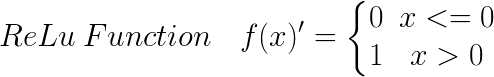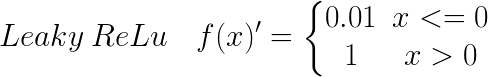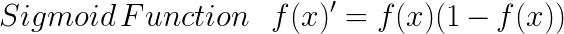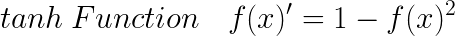```######################################
Step 4: Backward Propagation
######################################
def sigmoid_backward_propagation(dA,z):
sig=sigmoid(z)
dz = dA * sig * (1-sig)
return dz
def relu_backward_propagation(dA,z):
dz = np.array(dA,copy=True)
dz[z<=0]=0
return dz
def single_layer_backward_propagation(dA_cur,w_cur,b_cur,z_cur,A_prev,activation):
#Number of example
m=A_prev.shape
```# Part 1: Derivative for activation function
# Select activation function
if activation is 'sigmoid':
backward_activation_func = sigmoid_backward_propagation
elif activation is 'relu':
backward_activation_func = relu_backward_propagation
else:
raise Exception ('Not supported activation function')
# calculate derivative
dz_cur = backward_activation_func(dA_cur,z_cur)

# Part 2: Derivative for linear combination
dw_cur = np.dot(dz_cur,A_prev.T)/m
db_cur = np.sum(dz_cur,axis=1,keepdims=True)/m
dA_prev = np.dot(w_cur.T,dz_cur)

return dA_prev, dw_cur, db_cur
```
Test
dA_cur = - (np.divide(y,test_AL) - np.divide((1-y),(1-test_AL)))
dA_prev,dw_cur,db_cur=single_layer_backward_propagation(dA_cur,test_parameters[‘w2’],test_parameters[‘b2’],
caches[‘z2’],caches[‘a1’],‘sigmoid’)
print(dw_cur)
print(db_cur)
print(dA_prev)
def full_backward_propagation(AL,y,caches,parameters):
```grads={}
Length = len(caches)//2
m = AL.shape
y = y.reshape(AL.shape)

# Step 1: Derivative for cost function
dA_cur = - (np.divide(y,AL) - np.divide((1-y),(1-AL)))

# Step 2: Sigmoid backward propagation for N layer
w_cur = parameters['w'+str(Length)]
b_cur = parameters['b'+str(Length)]
z_cur = caches['z'+str(Length)]
A_prev = caches['a'+str(Length-1)]

dA_prev, dw_cur, db_cur = single_layer_backward_propagation(dA_cur,w_cur,b_cur,z_cur,A_prev,'sigmoid')

# Step 3: relu backward propagation for 1:(N-1) layer
for i in reversed(range(1,Length)):
dA_cur = dA_prev
w_cur  = parameters['w'+str(i)]
b_cur  = parameters['b'+str(i)]
z_cur  = caches['z'+str(i)]
A_prev = caches['a'+str(i-1)]

dA_prev, dw_cur, db_cur = single_layer_backward_propagation(dA_cur,w_cur,b_cur,z_cur,A_prev,'relu')

```
Test
```

Similar to forward propagation. First, you need to create a function for derivative of sigmoid and ReLU. Then define a function for single layer backward propagation, which calculates dWdB, and dA_prevdA_prev will be used as input for backward propagation for previous hidden layer. Finally, function created in the previous step is nested into the function called full backward propagation. To align with forward propagation, first N-1 hidden layers use ReLU function and last hidden layer or output layer uses sigmoid function. You can modify the code and add more activation function as you wish. Save dW and dB into another caches, which will be used to update parameters.

Here is the function output on testing data.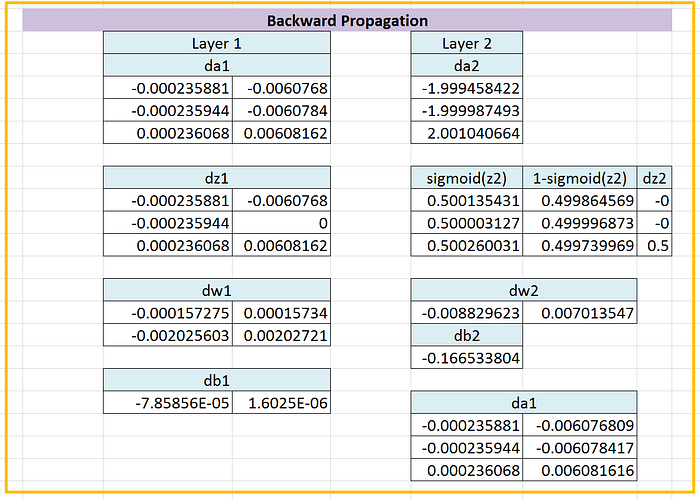Table 6: Backward Propagation Testing Result

# Update Parameters

```########################################
Step 5 Update parameters
########################################
Length = len(parameters)//2
```for i in (range(1,Length+1)):

return parameters
```
test
print(test_parameters_update[‘w1’])
print(test_parameters_update[‘b1’])
print(test_parameters_update[‘w2’])
print(test_parameters_update[‘b2’])
```

Once gradients are calculated from backward propagation, update the current parameters by learning rate * gradients. Then updated parameters are used in a new round of forward propagation.

Here is the function output on testing data.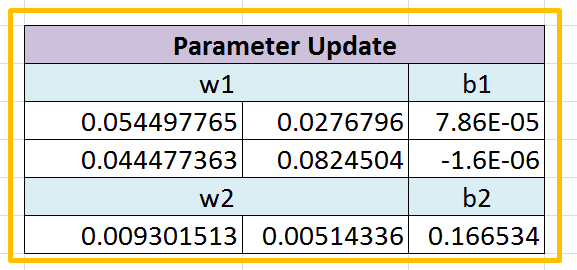Table 7: Parameter Update Testing Result

Explanation for gradient descent can be seen in my blog.

# Stack functions together

```#######################################
Step 6: Train Nerual Network Model
#######################################
def train_model(X,y,epoch,layer_dim,learning_rate):
# Store historical cost
cost_history = []
accuracy_history = []
epoches=[]
# Step 1: Initialize parameters
parameters = initialize_parameters(layer_dim)
```for i in range(1,epoch):
# Step 2: Forward propagation
AL, caches = full_forward_propagation(X,parameters)

# Step 3: Calculate and store cost
cost = cost_function(AL,y)
cost_history.append(cost)

accuracy =get_accuracy(AL,y)
accuracy_history.append(accuracy)

epoches.append(i)
# Step 4: Backward propagation

# Step 5: Update parameters

if(i % 100 ==0):
print('i='+str(i)+' cost = ' + str(cost))
print('i='+str(i)+' accuracy = '+str(accuracy))
#print(parameters)

return parameters,cost_history, accuracy_history, epoches
```
```

To train a neural network model, functions created in previous steps are stacked together. Summary of functions used is provided in the table below.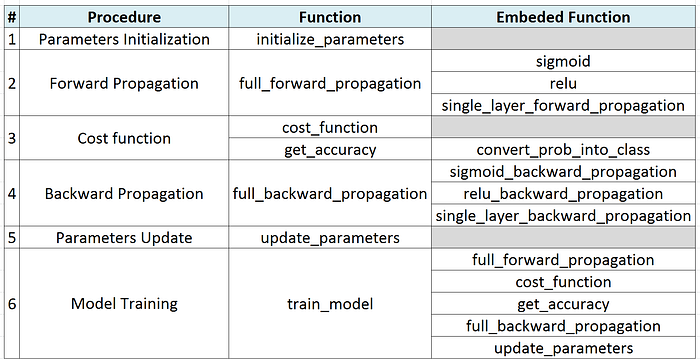Table 8: Functions Summary

# Run Model

```###############################
Create Random Dataset
###############################
N_SAMPLES = 1000
X, y = make_moons(n_samples = N_SAMPLES, noise=0.2, random_state=100)
###############################
Run Algorithm
###############################
test_parameters,test_cost,test_accuracy,test_epoches=train_model(X.T, y, 10000, [2,25,100,100,10,1],0.01)
```

First use make_moons function create two interleaving half circles data. Visualization of data is provided below.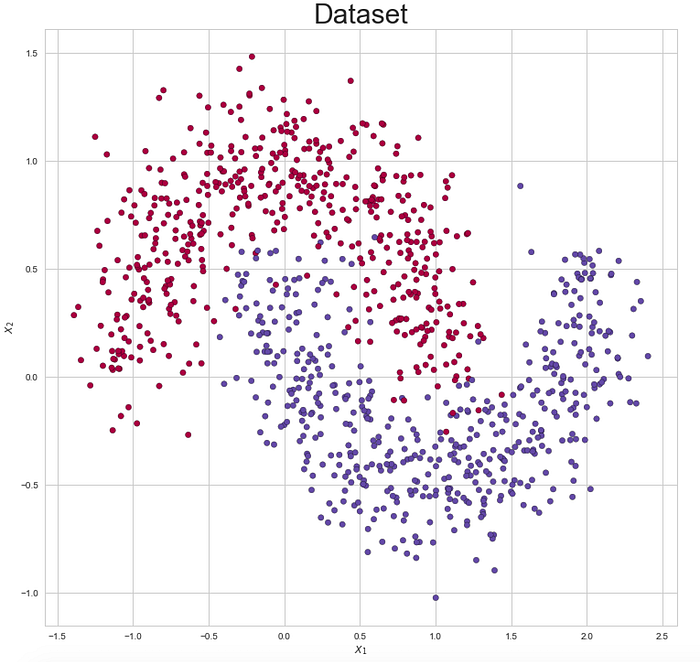Figure 4: Training Data

Then run the function to train a neural network model. Training process is visualized in the figures below. Cost converges after 8000 epochs and model accuracy rate converge to 0.9.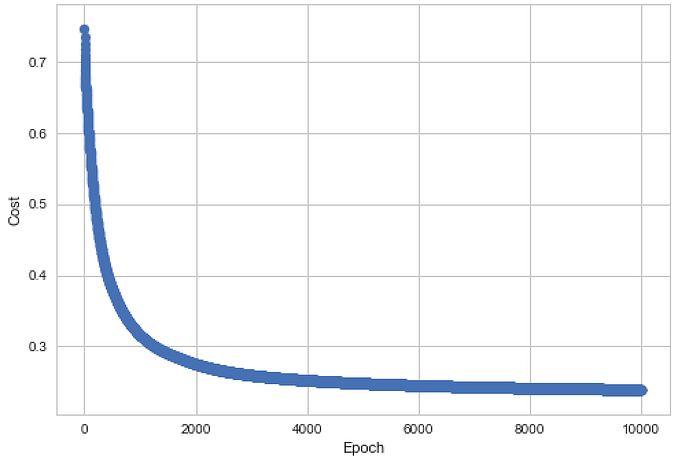Figure 5: Cost over Time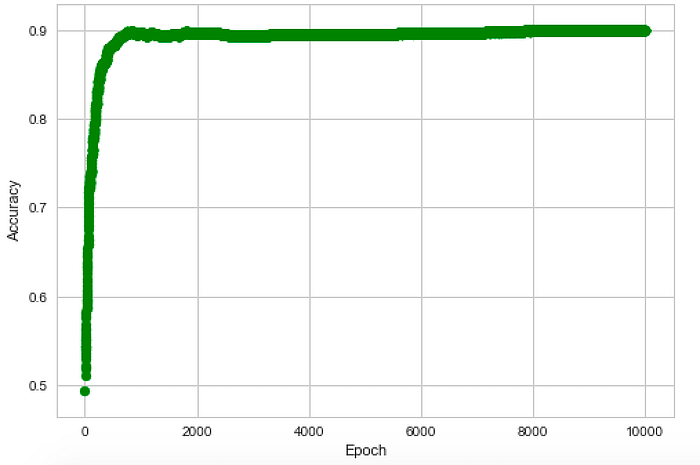Figure 6: Accuracy over Time

# Next Step

From figure 5 and 6, there is potential overfitting problem. You can use methods including early stop, dropout and regularization to remediate this issue. You can play with model by adding other activation functions besides ReLU and sigmoid function. Batch gradient descent is used in this blog, but there are many improved gradient descent algorithms such as Momentum, RMSprop, Adam and so on.

# Summary

Though taking online courses and read relevant chapters in the book before, not until I hands on the coding and writing blog by myself, I fully understood this fancy method. As an old saying goes, teaching is the best way to learn. Hope you can benefit by reading this blog. Please read my other blogs if you have interest.

============================================

#python #numpy #deep-learning #machine-learning

## Buddha Community1564563164

Endorse1619518440

## top 30 Python Tips and Tricks for Beginners

Welcome to my Blog , In this article, you are going to learn the top 10 python tips and tricks.

### 8) Check The Memory Usage Of An Object.

#python #python hacks tricks #python learning tips #python programming tricks #python tips #python tips and tricks #python tips and tricks advanced #python tips and tricks for beginners #python tips tricks and techniques #python tutorial #tips and tricks in python #tips to learn python #top 30 python tips and tricks for beginners1619510796

## Lambda, Map, Filter functions in python

Welcome to my Blog, In this article, we will learn python lambda function, Map function, and filter function.

Lambda function in python: Lambda is a one line anonymous function and lambda takes any number of arguments but can only have one expression and python lambda syntax is

Syntax: x = lambda arguments : expression

Now i will show you some python lambda function examples:

#python #anonymous function python #filter function in python #lambda #lambda python 3 #map python #python filter #python filter lambda #python lambda #python lambda examples #python map1624752180

## How to build your own Neural Network from scratch in Python

When discussing neural networks, most beginning textbooks create brain analogies. I can define the new neural networks simply as a mathematical function that translates a certain entry to the desired performance without going into brain analogies.

You may note that the weights W and biases b are the only variables in the equation above affecting the output of a given value. The strength of predictions naturally establishes the correct values for weights and biases. The weight and bias adjustment procedure of the input data is known as neural network training.

#neural-networks #artificial-intelligence #python #programming #technology #how to build your own neural network from scratch in python1623135499

## No Code introduction to Neural Networks

### The simple architecture explained

Neural networks have been around for a long time, being developed in the 1960s as a way to simulate neural activity for the development of artificial intelligence systems. However, since then they have developed into a useful analytical tool often used in replace of, or in conjunction with, standard statistical models such as regression or classification as they can be used to predict or more a specific output. The main difference, and advantage, in this regard is that neural networks make no initial assumptions as to the form of the relationship or distribution that underlies the data, meaning they can be more flexible and capture non-standard and non-linear relationships between input and output variables, making them incredibly valuable in todays data rich environment.

In this sense, their use has took over the past decade or so, with the fall in costs and increase in ability of general computing power, the rise of large datasets allowing these models to be trained, and the development of frameworks such as TensforFlow and Keras that have allowed people with sufficient hardware (in some cases this is no longer even an requirement through cloud computing), the correct data and an understanding of a given coding language to implement them. This article therefore seeks to be provide a no code introduction to their architecture and how they work so that their implementation and benefits can be better understood.

Firstly, the way these models work is that there is an input layer, one or more hidden layers and an output layer, each of which are connected by layers of synaptic weights¹. The input layer (X) is used to take in scaled values of the input, usually within a standardised range of 0–1. The hidden layers (Z) are then used to define the relationship between the input and output using weights and activation functions. The output layer (Y) then transforms the results from the hidden layers into the predicted values, often also scaled to be within 0–1. The synaptic weights (W) connecting these layers are used in model training to determine the weights assigned to each input and prediction in order to get the best model fit. Visually, this is represented as:

#machine-learning #python #neural-networks #tensorflow #neural-network-algorithm #no code introduction to neural networks1602968400

## Python Tricks Every Developer Should Know

Python is awesome, it’s one of the easiest languages with simple and intuitive syntax but wait, have you ever thought that there might ways to write your python code simpler?

In this tutorial, you’re going to learn a variety of Python tricks that you can use to write your Python code in a more readable and efficient way like a pro.

### Let’s get started

Swapping value in Python

Instead of creating a temporary variable to hold the value of the one while swapping, you can do this instead

``````>>> FirstName = "kalebu"
>>> LastName = "Jordan"
>>> FirstName, LastName = LastName, FirstName
>>> print(FirstName, LastName)
('Jordan', 'kalebu')
``````

#python #python-programming #python3 #python-tutorials #learn-python #python-tips #python-skills #python-development